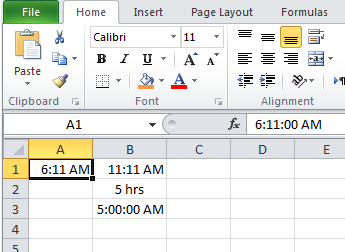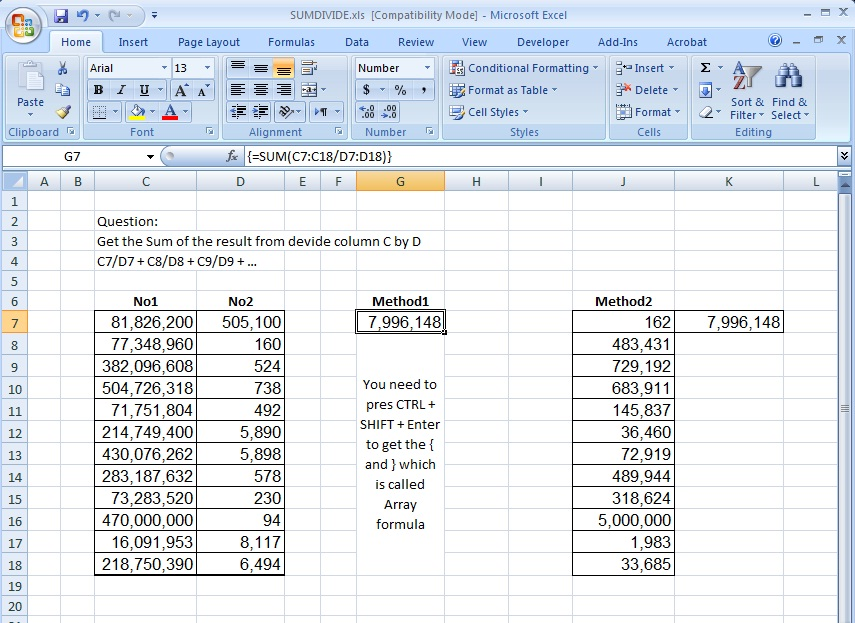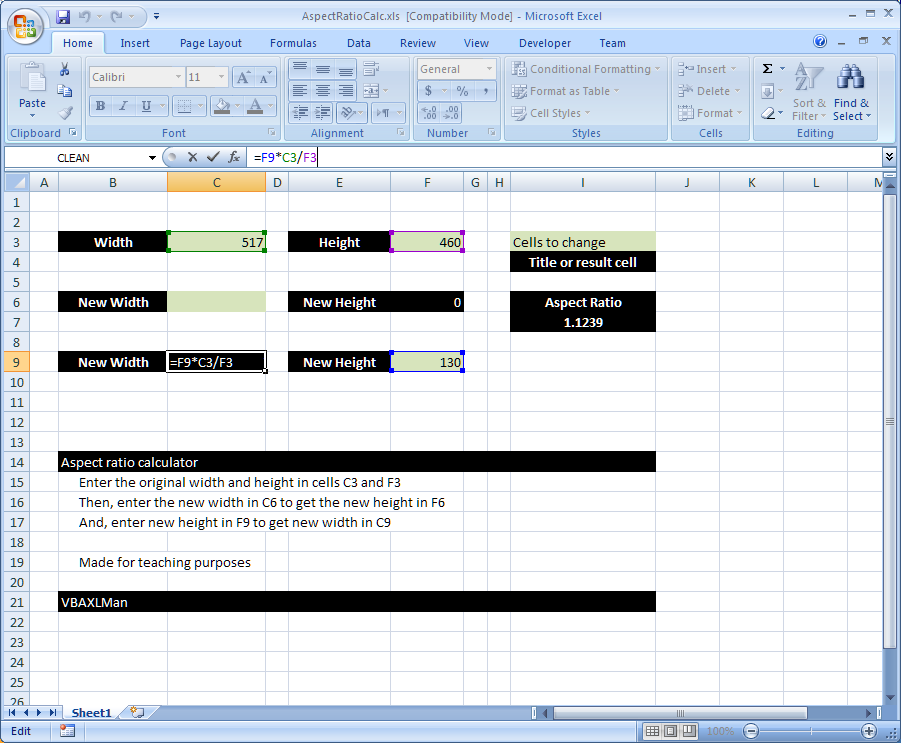## Calculate hours between two cellsWe needed that several times in the past Then I found it in an old file in my laptop, thought to share it for others to help So, when A1 …

## Sum of divide – Array formulaThis is the answer to an old question: Here is the story behind it: I had a client who calls me every now and then asking the same question: ” …

## Standard math to calculate Aspect RatioHere is a simple formula that uses standard math functions (+ , – , / and *) to calculate the aspect ratio I often need some simple formula like this …